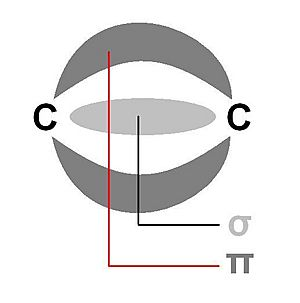# Double bond facts for kids

Kids Encyclopedia FactsA diagram of the hydrocarbon hex-3-ene, containing a carbon-carbon double bond. Highlighted in red, the way to draw a double bond in skeletal formula.

A double bond in chemistry is when two chemical elements are joined together in a chemical bond and share four bonding electrons instead of the usual two. The most common double bond is between two carbon atoms, and can be found in alkenes. There are many types of double bonds, such as in a carbonyl group with a carbon atom and an oxygen atom. Other common double bonds are found in azo compounds (N=N), imines (C=N) and sulfoxides (S=O). In skeletal formula the double bond is shown as two parallel lines (=) between the two joined atoms. When this formula is printed, the equals sign is used.A simplified diagram, showing where the sigma bond and the pi bond exist between two double bonded carbon atoms.

Double bonds are stronger than single bonds and double bonds are also shorter. The bond order is two. Double bonds are also electron-rich, which makes them more reactive.

The two pairs of electrons involved in the bond do not behave in exactly the same way. One pair of electrons forms a stronger sigma bond, noted by σ and drawn as a straight line between the nuclei of the bonding atoms. While the other pair form a pi bond, π, drawn as two cloud-like areas where the electrons exist, as orbitals.

## Images for kidsDouble bond Facts for Kids. Kiddle Encyclopedia.